DraftKings 2019 AAC Odds
+190
1.9 to 1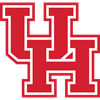Houston
28.5% implied probability

+350
3.5 to 1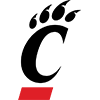Cincinnati
18.4% implied probability

+400
4 to 1Wichita State
16.5% implied probability

+650
6.5 to 1Memphis
11.0% implied probability

+650
6.5 to 1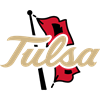Tulsa
11.0% implied probability

+1400
14 to 1Connecticut
5.5% implied probability

+2500
25 to 1SMU
3.2% implied probability

+5000
50 to 1Central Florida
1.6% implied probability

+5000
50 to 1Temple
1.6% implied probability

+8000
80 to 1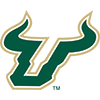South Florida
1.0% implied probability

+10000
100 to 1East Carolina
0.8% implied probability

+10000
100 to 1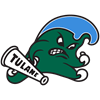Tulane
0.8% implied probability